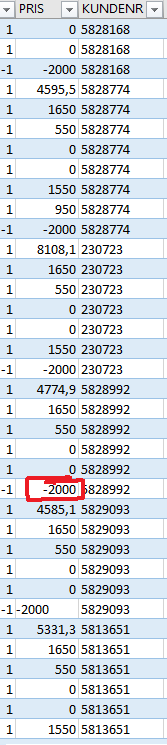# Turning a negative value to positive

Hello i am using a excel sheet ( thats a sql ) to retreive some numbers. Only problem its when i come to the negative number it only gives me 0. I have tries doing System.text.RegularExpressions.Regex.Replace(“-”+price_variable,“-”,“”)

but still spits out 0. How can i make a function that see if its a -number then it turns it into a positive and assign it to a var?=

@langsem Math.Abs(CDbl(“-1”)) will give you 1. In the same way you can try for your Purpose

1 Like

shall i change out the number one with my variable?

@langsem Yes, You can Always try and Checkstill gives me 0

@langsem Can you tell me what is the Number you are trying to Convert ?

Worked now, could be a bug i guess

Can you explain me how can we achieve this
I need to change - to + and + to -

Price = math.abs(Price("-1).tostring)
It is correct
And then price variable should be which datatype

Thanks @supermanPunch this was helpful in converting -1,234 to 1,234, Can you help me on how to convert 1,234 to -1,234(Positive value to Negative value)